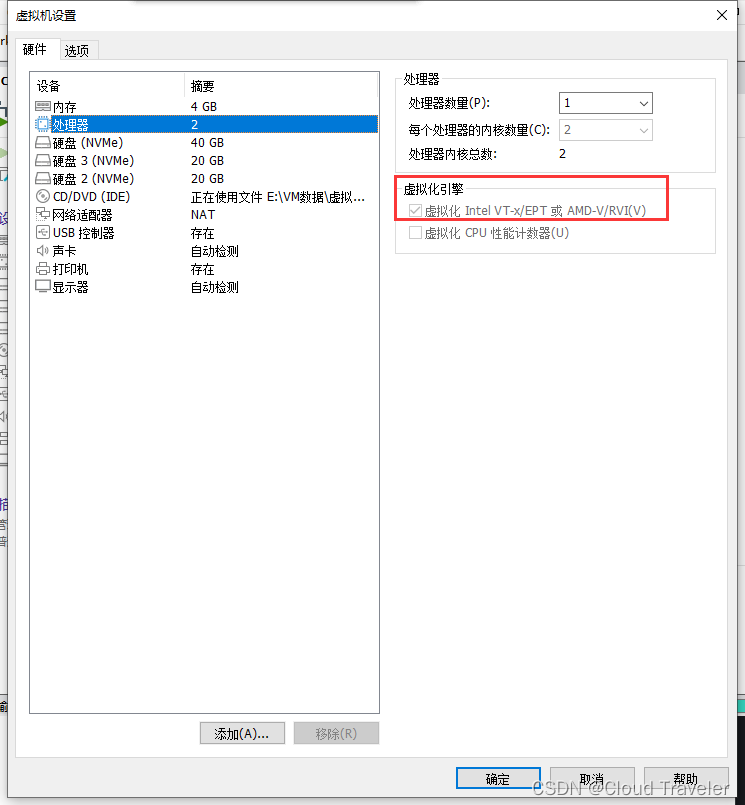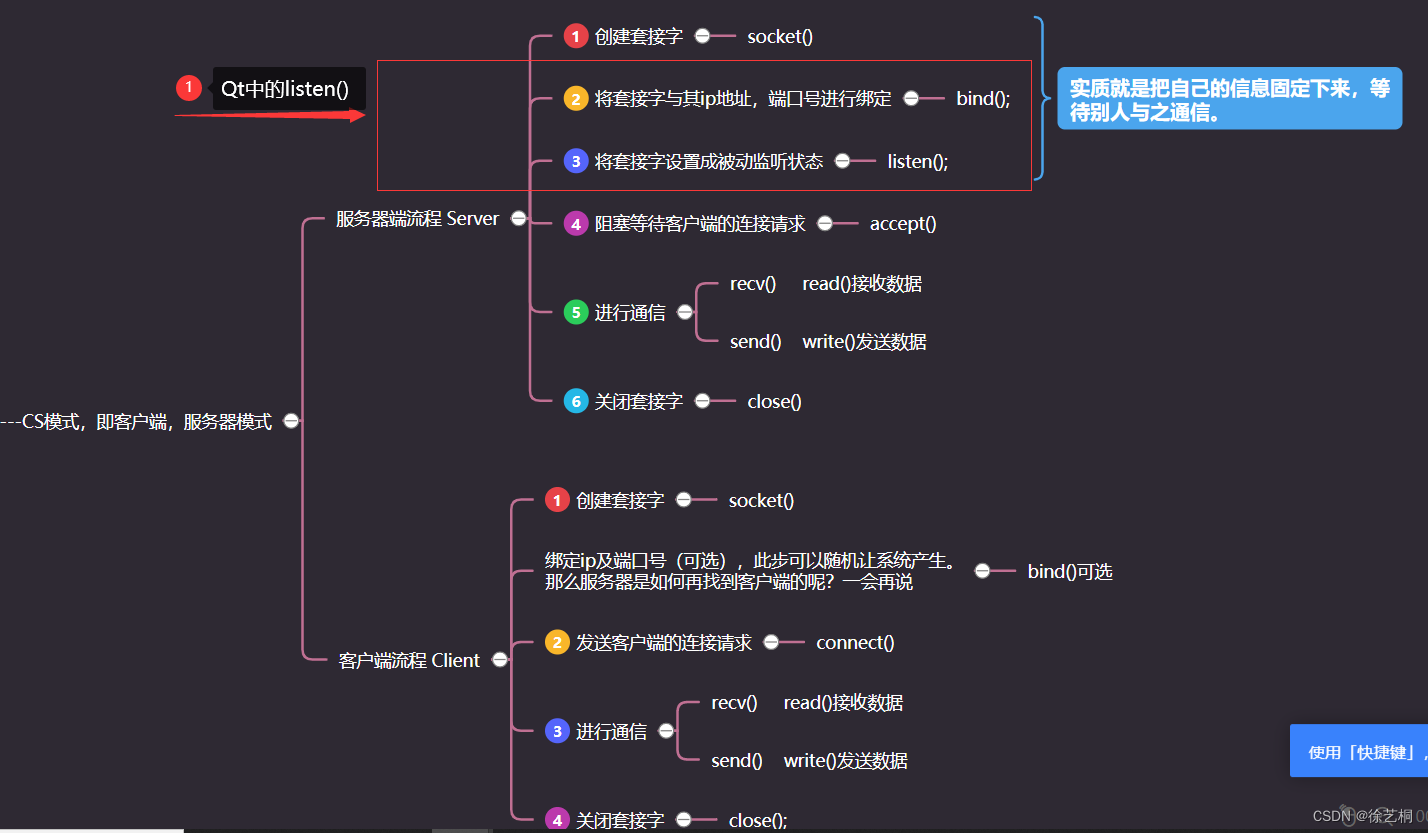# 无涯教程-JavaScript - IMCOS函数

## 描述

IMCOS函数以x + yi或x + yj文本格式返回复数的余弦。

## 语法

IMCOS (inumber)


## 争论

Argument 描述 Required/Optional
Inumber A Complex Number for which you want the cosine. Required

## Notes

• Excel中的复数仅存储为文本。

• 当将格式为" a + bi"或" a + bj"的文本字符串提供给Excel的内置复数函数之一时,这被解释为复数。

• 复数函数可以接受一个简单的数值,因为它等效于虚数等于0的复数。

• 使用COMPLEX将实系数和虚系数转换为复数。

• 复数的余弦为-

$$\cos(x + yi)= \cos(x)\cosh(y)-\sin(x)\sinh(y)i$$

• 如果inumber不被识别为复数,则IMCOS返回#NUM!错误。

• 如果inumber是逻辑值,则IMCOS返回#VALUE!错误。

## 适用性

Excel 2007,Excel 2010,Excel 2013,Excel 2016

## ExampleJavaScript 中的 IMCOS函数 - 无涯教程网无涯教程网提供描述IMCOS函数以x + yi或x + yj文本格式返回复数的余弦。 语法 IMCOS (inumber) 争论...https://www.learnfk.com/javascript/advanced-excel-engineering-imcos-function.html文章来源地址:https://www.uudwc.com/A/jrZaj/